# Matchings

Relevant articles: , , , .

# Matchings

The graphs considered in this section are$k$-matchings. A$k$-matching is a graph consisting of$k$ vertex disjoint edges and is denoted by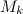$M_k$. The spectrum problem has been completely settled for matchings, see Theorem 1 below.

## Spectrum Results

Theorem 1 is a consequence of results in , ,  or .

Theorem 1

Let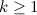$k\geq 1$. There exists an$M_k$-design of order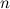$n$ if and only if

•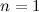$n=1$ or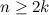$n\geq 2k$; and
•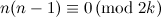$n(n-1)\equiv 0 \,({\rm mod \ }2k)$.

## References

1. 1.0 1.1 Alon, N. A note on the decomposition of graphs into isomorphic matchings, Acta Math. Hungar. 42, 221–223 (1983).
2. 2.0 2.1 de Werra, D. On some combinatorial problems arising in scheduling, CORS J. 8, 165–175 (1970).
3. 3.0 3.1 Ellingham, M. N. and Wormald, N. C. Isomorphic factorization of regular graphs and 3-regular multigraphs, J. London Math. Soc. (2), 37, 14–24 (1988).
4. 4.0 4.1 McDiarmid, C. J. H. The solution of a timetabling problem, J. Inst. Math. Appl. 9, 23–34 (1972).